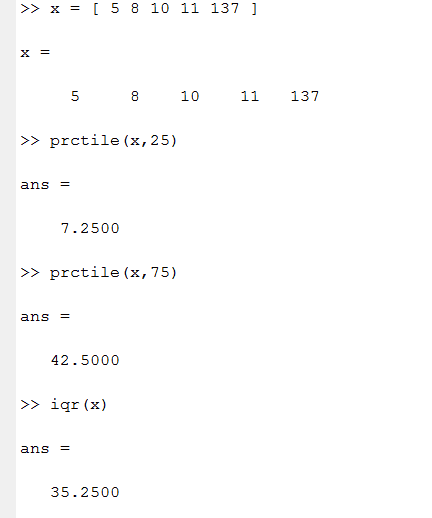# {statics} Kvartil (quartiles) why I didnt get right solution ?

## Homework Statement

On this vector I didnt get the right answer:

x = [ 5 8 10 11 137 ] [/B]
q3: i = (p/100)*n = (75/100)*5 = 3.75 >> 4th in vector is 11
q1: i = (25/100)*5 = 1.25 >> 2nd is 8

in MATLAB is solutionharuspex